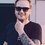# Again Doubt in titrations!

An aqueous solution containing 0.15 g KIO3 ( formula weight =220.0) was less treated with an excess of KI solution. The solution was acidified with HCl . The liberated I2 consumed 50 ml of thiosulphate solution to decolorize the blue starch iodine complex. Please help me write my paper how calculate the molarity of Na2S2O3 ??

My solution is Redox change IO3- ---> I2 2IO3- + 12H+ + 10e- = I2 + 6H2O n factor of KIO3 = 5 Eq wt of KIO3 = 214/5 Gm eq of KIO3 = 0.10/(214/5) = Gm eq of I2 Let the molarity of Na2S2O3 = x N factor of Na2S2O3 = 1 (0.10 x 5)/(214) = (45/1000) x (x) x 1 or x = 0.052M But the solution is 0.062M What is wrong in my solution.Note by Nick Yavorski
1 year, 7 months ago

This discussion board is a place to discuss our Daily Challenges and the math and science related to those challenges. Explanations are more than just a solution — they should explain the steps and thinking strategies that you used to obtain the solution. Comments should further the discussion of math and science.

When posting on Brilliant:

• Use the emojis to react to an explanation, whether you're congratulating a job well done , or just really confused .
• Ask specific questions about the challenge or the steps in somebody's explanation. Well-posed questions can add a lot to the discussion, but posting "I don't understand!" doesn't help anyone.
• Try to contribute something new to the discussion, whether it is an extension, generalization or other idea related to the challenge.

MarkdownAppears as
*italics* or _italics_ italics
**bold** or __bold__ bold
- bulleted- list
• bulleted
• list
1. numbered2. list
1. numbered
2. list
Note: you must add a full line of space before and after lists for them to show up correctly
paragraph 1paragraph 2

paragraph 1

paragraph 2

[example link](https://brilliant.org)example link
> This is a quote
This is a quote
    # I indented these lines
# 4 spaces, and now they show
# up as a code block.

print "hello world"
# I indented these lines
# 4 spaces, and now they show
# up as a code block.

print "hello world"
MathAppears as
Remember to wrap math in $$ ... $$ or $ ... $ to ensure proper formatting.
2 \times 3 $2 \times 3$
2^{34} $2^{34}$
a_{i-1} $a_{i-1}$
\frac{2}{3} $\frac{2}{3}$
\sqrt{2} $\sqrt{2}$
\sum_{i=1}^3 $\sum_{i=1}^3$
\sin \theta $\sin \theta$
\boxed{123} $\boxed{123}$

Sort by:

Your decision is true. You tried to do it. The next time you do not waste your nerves and avoid stress, use a special service. I use the writing service. There I get a college coursework help and help with writing an essay. You can use this to get your paper's writing

- 1 year, 4 months ago